## General Question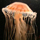# The amount of X's that can fit inside Y?

Asked by dotlin (419) August 14th, 2010

Normally when we work out how many of X can fit into Y we divide Y by X. Is this the right approach when maybe X can’t be perfectly stacked flush next to another X such as with two circles?

If Y was a square and X is a circle should we divide Y by two times the radius of X?

Now imagine Y is a circle and X are also circles what’s the best approach at working out how many X’s fit into Y.

Are there some rules to follow when the shapes become more random?

Observing members: 0Composing members: 0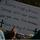Take the area of the outside shape and divide it by the area of the inside shape. That will tell you how many inside shapes fit into the bigger shape.

BarnacleBill (16070)“Great Answer” (2) Flag as…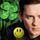First, are you talking about volume or area or a line? (I will assume you mean area).

You need to find the formula for the area for each of the shapes you are matching.
The area of a rectangle is base x height. A=b x h
The area of a circle is Pi x radius squared. A= 3.1416 x r^2
IThen you divide the two areas
Let’s do an example How many 2 cm diameter circles (4 cm diameter) will fit into a rectangle of 8 cm by 10 cm?
Figure out the area of the rectangle A=bxh ; A=8×10 =80 sq cm
Now figure the area of the circle. A= 3.1416×2^2 = 12.56 sq cm
Now divide 80/12.56 = 6.36 so the area of 6.36 circles will fit in the rectangle.

If you are doing volume you follow the same rules but use the formulas for volume.
There are many math sites that list the formulas for area and volume.

LuckyGuy (36349)“Great Answer” (2) Flag as…@worriedguy
But surely it will come out wrong because it will take the gaps between the circles as if more circles could fit in there when you can’t.

dotlin (419)“Great Answer” (0) Flag as…With a circle pi*r^2 r=2 the area will be 12.56

Now a square with sides 3.544 area will also come out to 12.56 but you can fit more of these in because there will be no gaps.

dotlin (419)“Great Answer” (0) Flag as…@dotlin, the question requires you to work with two dimensional shapes. A circle is a defined thing. You will have leftover area, but the area itself is not a circle. You could fit circles with smaller radiuses into the leftover space.

BarnacleBill (16070)“Great Answer” (0) Flag as…@BarnacleBill
Pardon?

dotlin (419)“Great Answer” (0) Flag as…A square has sides of 2. Area = 4. A circle has a radius of 1. Area = 3.14. If you divide 4 by 3.14 you get 1.274. You can fit 1 whole circle into the square, and .274 of a circle with a radius of 1 into the remaining area of the square. If you want whole circles in the remaining area, they must be circles with smaller radiuses.

BarnacleBill (16070)“Great Answer” (0) Flag as…I mean here’s an image I made http://yfrog.com/49shapespj

The circles had diameter of 4 and the squares 3.54 look you will be able to fit more squares in if the larger square was bigger.

dotlin (419)“Great Answer” (0) Flag as…I don’t think you’re understanding me in real life how many of these circles could you fit inside this bigger circles? you can’t fit .274 of a circle in there WTF, I get it mathematically you can but I mean without cutting anyor anything like that how many whole circle could we fit in there.

dotlin (419)“Great Answer” (0) Flag as…Correct. Sometimes you have area that you can’t use when you’re working with two dimensional shapes. An example of what you’re saying is sewing. When you go to sew, you purchase fabric in a rectangle. You go to cut out your pattern pieces, they don’t fit exactly and you end up with leftover scraps of fabric from between the pattern pieces. You don’t use it all; you have waste.

BarnacleBill (16070)“Great Answer” (0) Flag as…@BarnacleBill
OK so reread my questions and how do you work these out but with how many whole shapes could you fit into Y without splitting the X’s?

dotlin (419)“Great Answer” (0) Flag as…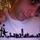I think that this turns out to be a hard question to answer in general. It probably falls under the auspices of packing problems. It generally requires some mathematical subtlety to deal answer this kind of question (if it can be answered at all). I don’t think there’s a general method like “just divide.” Cool question.

finkelitis (1907)“Great Answer” (2) Flag as…In general, figuring out how many of one shape will fit into another is a tricky matter, Sphere Packing Note that the article says that the best way to pack circles is to stagger the rows. One thing that you can do is to establish upper and lower bounds on how many of one shape can fit in another. If you divide areas, you have an upper bound. If you do a radius division, as you suggest, and then square that, you can get a lower bound. If the integer portion of both those numbers happens to be the same, then that would give you a unique answer.

LostInParadise (25488)“Great Answer” (1) Flag as…@finkelitis
Thanks.

dotlin (419)“Great Answer” (0) Flag as…Are there any places online where I can learn about packing problem apart from wiki?

Like videos or online books?

dotlin (419)“Great Answer” (0) Flag as…I didn’t realize you were discussing the packing problem. I misread your question and thoughtyou were concerned with the basic algebra. part. (That’s why I wrote out the words.)

Packing is a whole science.

LuckyGuy (36349)“Great Answer” (0) Flag as…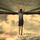Not sure if anyone has mentioned it, but you cannot determine it by volumes alone. The topography (not sure if this is the correct term to use in this context) also determines how much can be packed. For example a thin tube can theoretically hold the volume say 20 smarties, but perhaps it is too narrow to actually hold any in real life without distorting either object.

Assuming you like your smarties whole and not crushed up that is.

Ame_Evil (3041)“Great Answer” (0) Flag as…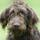Although I am an expert on fitting square pegs into round holes, this is too tempting not to weigh in.
From your question, my sense is that you want to pack round things into square boxes and want to see how many will fit. In addition to the dimensions etc, in the case of packing you also have to consider fragility. If you are packing round Christmas ornaments you have to allow for protective dividers. If you are packing BB’s (read BB gun—little round METAL spheres), there is no need for protective packing. So the nature of the packed object requires the addition of accounting for the space of any protective packaging required by the object.

Hence @worriedguy‘s observation that packing is a science. I would add that packing is an art!

Kayak8 (16440)“Great Answer” (0) Flag as…or